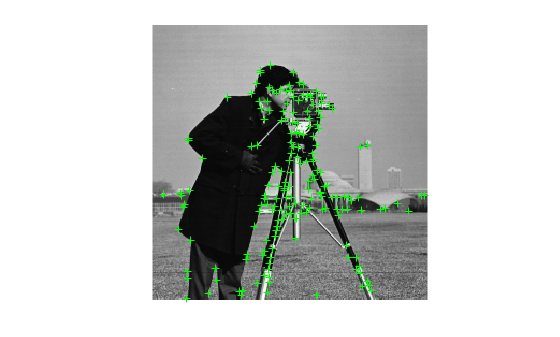# plot

## Syntax

``plot(points)``
``plot(points,ax)``

## Description

example

````plot(points)` plots points in the current axis.```
````plot(points,ax)` plots points in the specified axis.```

## Examples

collapse all

`I = imread("cameraman.tif");`

Detect corner features.

`featurePoints = detectHarrisFeatures(I);`

Plot feature image with detected features.

```imshow(I); hold on; plot(featurePoints);```## Input Arguments

collapse all

Points object,specified as an M-by-2 matrix of M number of [x y] coordinates, or as one of the point feature objects described in Point Feature Types. The object contains information about the feature points detected in the input image. To obtain points, use the appropriate detect function that pairs with the point feature type.

Handle to use for display. You can set the handle using `gca`.

## Version History

Introduced in R2011b